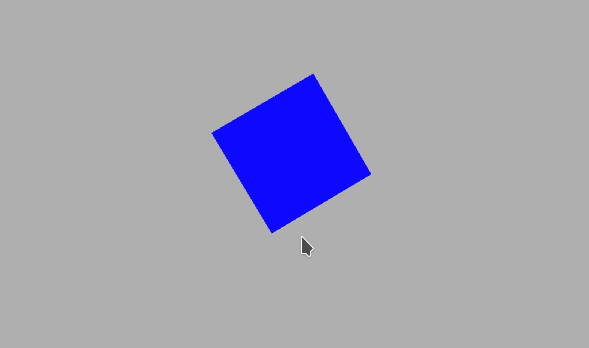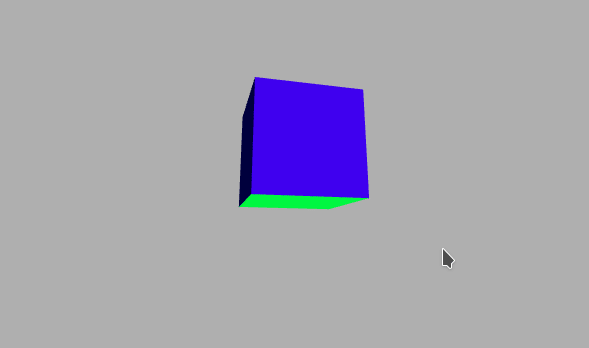Related Articles

# p5.js camera() Method

• Difficulty Level : Medium
• Last Updated : 04 Mar, 2021

The camera() function in p5.js is used to set the virtual camera’s position on a 3D sketch. This can be used to simulate the position of the camera as it would move around the scene making it possible to view objects from various angles.

The parameters of this function include setting the position for the camera, the center of the camera, and the vector which is pointing upside.

Syntax:

```camera([x], [y], [z], [centerX], [centerY], [centerZ],
[upX], [upY], [upZ])```

Parameters: This function accept nine parameters as mentioned above and described below:

• x: This is a number that denotes the camera position on x-axis.
• y: This is a number that denotes the camera position on y-axis.
• z: This is a number that denotes the camera position on z-axis.
• centerX: This is a number that denotes the x coordinate of the center of the sketch.
• centerY: This is a number that denotes the y coordinate of the center of the sketch.
• centerZ: This is a number that denotes the z coordinate of the center of the sketch.
• upX: This is a number that denotes the x component of direction ‘up’ from camera.
• upY: This is a number that denotes the y component of direction ‘up’ from camera.
• upZ: This is a number that denotes the z component of direction ‘up’ from camera.

Below examples illustrates the camera() function in p5.js:

Example 1: Set the view of the camera on the x-axis.

## Javascript

 `function` `setup() {``   ` `  ``createCanvas(600, 400, WEBGL);``}`` ` `function` `draw() {``   ` `  ``background(175);``   ` `  ``// Map the coordinates of the mouse``  ``// to the variable``  ``let cX = map(mouseX, 0,``               ``width, -200, 200);``   ` `  ``// Set the camera to the given coordinates``  ``camera(cX, 0, (height/2) / tan(PI/6),``         ``cX, 0, 0, 0, 1, 0);``   ` `  ``ambientLight(255);``   ` `  ``rotateZ(frameCount * 0.01);``  ``rotateX(frameCount * 0.03);``  ``rotateY(frameCount * 0.06);``  ``noStroke();``  ``normalMaterial();``   ` `  ``box(100, 100, 100);``}`

Output:Example 2: Set the view of the camera in a random direction every frame.

## Javascript

 `function` `setup() {``   ` `  ``frameRate(5);``  ``createCanvas(600, 400, WEBGL);``}`` ` `function` `draw() {``   ` `  ``background(175);``   ` `  ``let cX = random(-10,10);``  ``let cY = random(-10,10);``  ``let cZ = random(-10,10);``   ` `  ``camera(cX, cY,``         ``cZ+(height/2) / tan(PI/6),``         ``cX, 0, 0, 0, 1, 0);``   ` `  ``ambientLight(255);``   ` `  ``rotateX(frameCount * 0.1);``  ``rotateY(frameCount * 0.1);``   ` `  ``noStroke();``  ``normalMaterial();``   ` `  ``box(100, 100, 100);``}`

Output:Reference:https://p5js.org/reference/#/p5.Camera

My Personal Notes arrow_drop_up